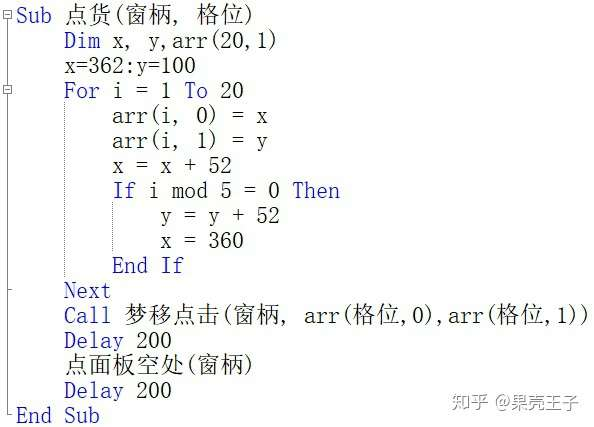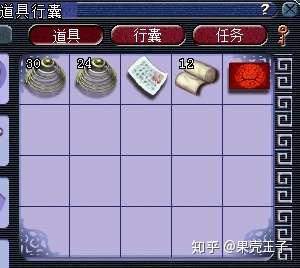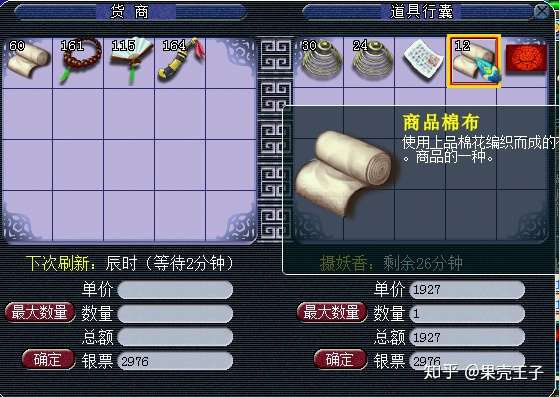1#
1. Sub 点货(窗柄, 格位)
2. Dim x, y,arr(20,1)
3. x=362:y=100
4. For i = 1 To 20
5. arr(i, 0) = x
6. arr(i, 1) = y
7. x = x + 52
8. If i mod 5 = 0 Then
9. y = y + 52
10. x = 360
11. End If
12. Next
13. Call 梦移点击(窗柄, arr(格位,0),arr(格位,1))
14. Delay 200
15. 点面板空处(窗柄)
16. Delay 200
17. End SubCall 梦移点击(窗柄, arr(格位,0),arr(格位,1))

 爱点哪里点哪里！点货的目的当然是为了卖货啦，爱跑商的同学一看便知！老板，你觉得呢？（此图为道具栏20格界面）（此图为跑商对话NPC弹出的交易框界面）